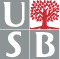# MAT 615 Topics in Algebraic Geometry

### Schedule:

TuTh 12:50pm-2:10pm Physics P129 (first class on 02/03)

### About the course

Curves - Geometry and moduli: The course aims to provide an introduction to moduli spaces of curves, deformation theory and Geometric Invariant Theory. Possible/tentative topics are the following:

• Curves: Riemann-Roch, Serre duality, Riemann-Hurwitz formula (crash course)
• Hyperelliptic curves, the canonical embedding, Clifford's theorem
• Curves of low genus. Rudiments of Brill-Noether theory.
• Parameter spaces, moduli spaces, Hilbert scheme
• Tangent space, dimension of the Hilbert scheme
• Deformation theory, Kodaira-Spencer maps
• Constructions of the moduli space of curves
• The ring of tautological classes
• Background on GIT
• The Deligne-Mumford compactification of the moduli space
• Moduli spaces of stable maps, quantum cohomology, Gromov-Witten invariants

### References

Basic references for the course are
• Moduli of Curves, J. Harris and I. Morrison, GTM 187, Springer
• Geometric invariant theory, D. Mumford, J. Fogarty, and F. Kirwan, 3rd ed.Springer
• Geometry of algebraic curves, Geometry of Algebraic Curves, E. Arbarello, M. Cornalba, Ph. Griffiths, J. Harris.
Among other useful references